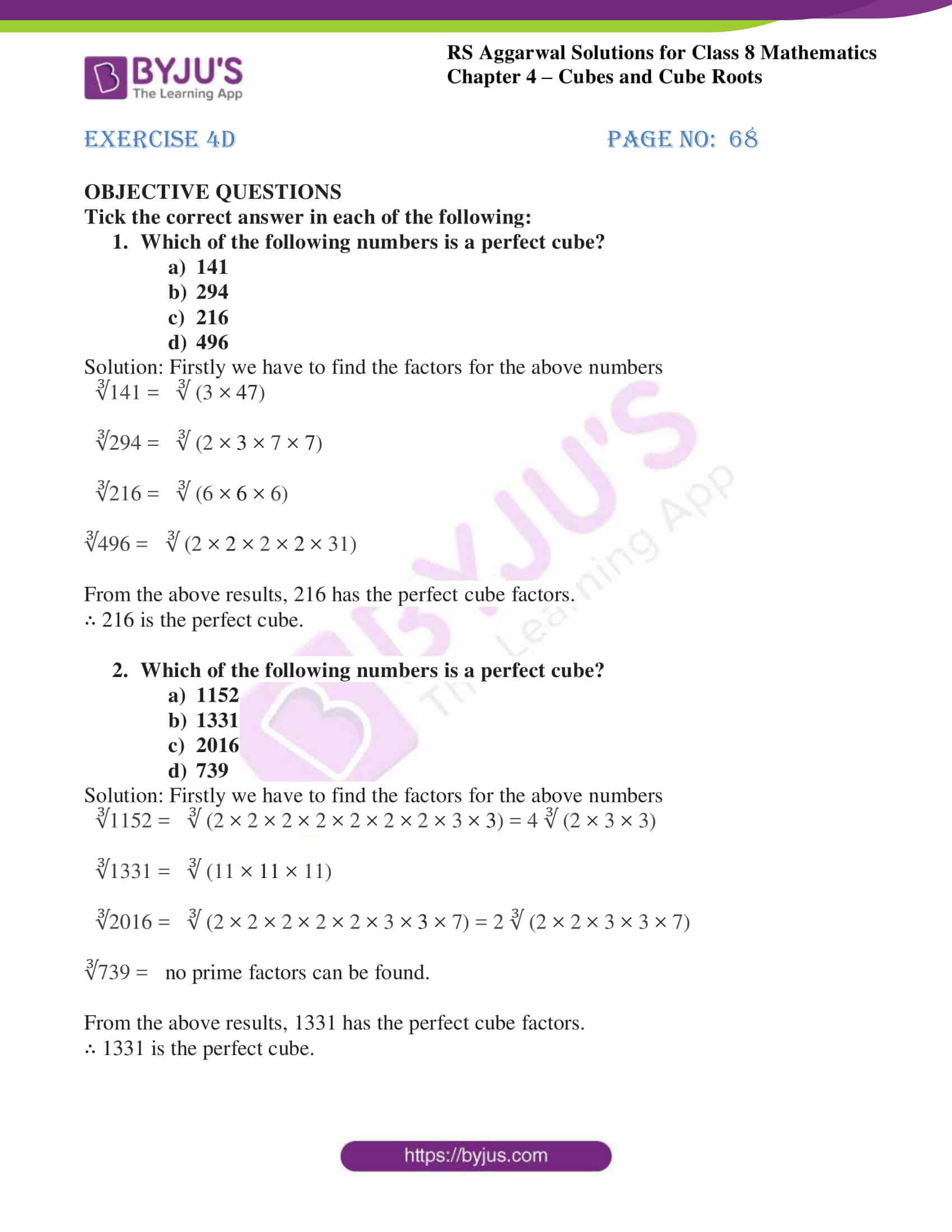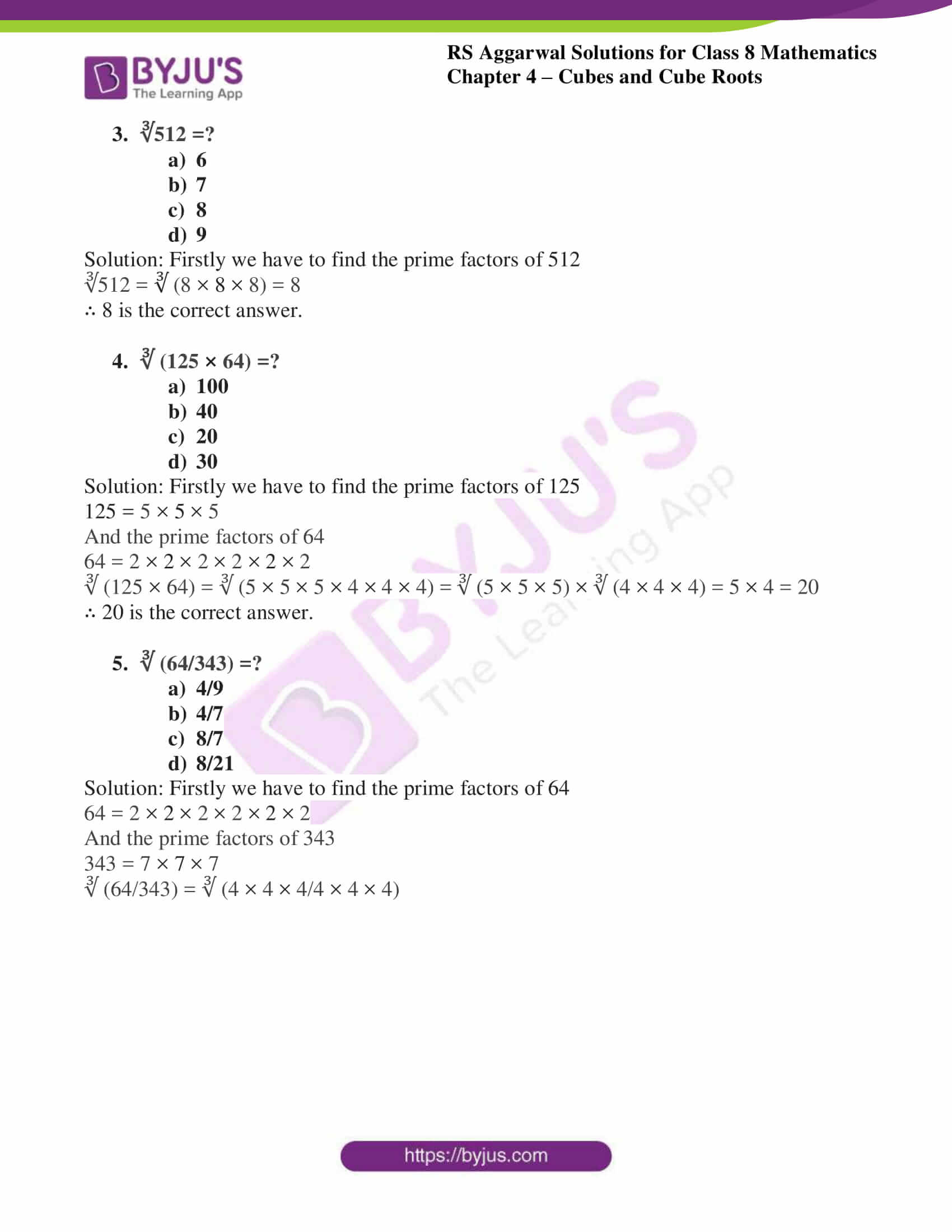# RS Aggarwal Solutions for Class 8 Chapter 4 - Cubes and Cube Roots Exercise 4D

Our experts have solved the RS Aggarwal Solutions to ensure that the students are thorough with their basic concepts by practising the solutions. Students can refer and download RS Aggarwal Solutions for Class 8 Maths Chapter 4- Exercise 4D, Cubes and Cube Roots from the links provided below.

In Exercise 4D of RS Aggarwal Class 8 Maths, we shall brief all the concepts covered in this Chapter 4 i.e. cube of a number, properties of cubes of numbers and methods for finding the cube.

## Download PDF of RS Aggarwal Solutions for Class 8 Maths Chapter 4- Exercise 4D### Access Answers to RS Aggarwal Solutions for Class 8 Maths Chapter 4- Cubes and Cube Roots Exercise 4D

Tick the correct answer in each of the following:

1. Which of the following numbers is a perfect cube?
a) 141
b) 294
c) 216
d) 496

Solution: Firstly we have to find the factors for the above numbers

∛141 = ∛ (3 × 47)

∛294 = ∛ (2 × 3 × 7 × 7)

∛216 = ∛ (6 × 6 × 6)

∛496 = ∛ (2 × 2 × 2 × 2 × 31)

From the above results, 216 has the perfect cube factors.

∴ 216 is the perfect cube.

2. Which of the following numbers is a perfect cube?
a) 1152
b) 1331
c) 2016
d) 739

Solution: Firstly we have to find the factors for the above numbers

∛1152 = ∛ (2 × 2 × 2 × 2 × 2 × 2 × 2 × 3 × 3) = 4 ∛ (2 × 3 × 3)

∛1331 = ∛ (11 × 11 × 11)

∛2016 = ∛ (2 × 2 × 2 × 2 × 2 × 3 × 3 × 7) = 2 ∛ (2 × 2 × 3 × 3 × 7)

∛739 = no prime factors can be found.

From the above results, 1331 has the perfect cube factors.

∴ 1331 is the perfect cube.

3. ∛512 =?
a) 6
b) 7
c) 8
d) 9

Solution: Firstly we have to find the prime factors of 512

∛512 = ∛ (8 × 8 × 8) = 8

∴ 8 is the correct answer.

4. ∛ (125 × 64) =?
a) 100
b) 40
c) 20
d) 30

Solution: Firstly we have to find the prime factors of 125

125 = 5 × 5 × 5

And the prime factors of 64

64 = 2 × 2 × 2 × 2 × 2 × 2

∛ (125 × 64) = ∛ (5 × 5 × 5 × 4 × 4 × 4) = ∛ (5 × 5 × 5) × ∛ (4 × 4 × 4) = 5 × 4 = 20

∴ 20 is the correct answer.

5. ∛ (64/343) =?
a) 4/9
b) 4/7
c) 8/7
d) 8/21

Solution: Firstly we have to find the prime factors of 64

64 = 2 × 2 × 2 × 2 × 2 × 2

And the prime factors of 343

343 = 7 × 7 × 7

∛ (64/343) = ∛ (4 × 4 × 4/4 × 4 × 4)

## RS Aggarwal Solutions for Class 8 Maths Chapter 4- Exercise 4D

Exercise 4D of RS Aggarwal Class 8, Cubes and Cube Roots contains advance concepts related to Cubes. This exercise mainly deals with the objective questions related to the concepts covered in Chapter 4.

The RS Aggarwal Solutions can help the students practice diligently while learning the fundamentals as it provides all the answers to the questions from the RS Aggarwal textbook. Students should practice RS Aggarwal Solutions rigorously to understand how the concepts are solved. Practising as many times as possible helps in time management skills and also enhances the confidence level to achieve high marks.